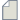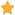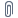###Author Topic: Strange restart from Checkpoint: Device calculation  (Read 28 times)

0 Members and 1 Guest are viewing this topic.

####Dongzhe

• New ATK user
•• Posts: 4
• Country:• Reputation: 0##### Strange restart from Checkpoint: Device calculation
« on: September 13, 2021, 06:38 »
Dear AKT experts,
My calculation has been stopped after 20 interactions for the device calculation (due to the exceed of #SBATCH --time), and I have saved properly the checkpoint file. Please see the convergence information as below:

--------------------- First run -----------------------
Left electrode
|   0 E = -45.1889 dE =  1.381850e+01 dH =  3.882496e-01                       |
|   1 E = -37.9049 dE =  7.283987e+00 dH =  3.257146e-01                       |
|   2 E = -38.5402 dE =  6.352730e-01 dH =  4.479190e-02                       |
|   3 E = -37.9072 dE =  6.330154e-01 dH =  1.841077e-02                       |
|   4 E = -38.1703 dE =  2.631561e-01 dH =  1.666323e-02                       |
|   5 E = -38.1205 dE =  4.986235e-02 dH =  1.686951e-03                       |
|   6 E = -38.1647 dE =  4.425834e-02 dH =  1.673110e-03                       |
|   7 E = -38.1395 dE =  2.526105e-02 dH =  1.226150e-03                       |
|   8 E = -38.1422 dE =  2.690740e-03 dH =  7.125102e-05                       |
Right electrode
|   0 E = -45.1889 dE =  1.381850e+01 dH =  3.882525e-01                       |
|   1 E = -37.9048 dE =  7.284075e+00 dH =  3.257171e-01                       |
|   2 E = -38.5401 dE =  6.352944e-01 dH =  4.479243e-02                       |
|   3 E = -37.9071 dE =  6.330298e-01 dH =  1.841124e-02                       |
|   4 E = -38.1702 dE =  2.631623e-01 dH =  1.666332e-02                       |
|   5 E = -38.1204 dE =  4.987045e-02 dH =  1.687070e-03                       |
|   6 E = -38.1646 dE =  4.426331e-02 dH =  1.673284e-03                       |
|   7 E = -38.1394 dE =  2.526172e-02 dH =  1.226157e-03                       |
|   8 E = -38.1421 dE =  2.690785e-03 dH =  7.126149e-05                       |
Device
|   0 E = -2200.65 dE =  5.196342e+02 dH =  2.766877e+01                       |
|   1 E = -2313.09 dE =  1.124387e+02 dH =  9.664825e+01                       |
|   2 E =  -2469.3 dE =  1.562185e+02 dH =  1.690583e+01                       |
|   3 E = -2394.04 dE =  7.526550e+01 dH =  2.487792e+01                       |
|   4 E =  -2357.3 dE =  3.673632e+01 dH =  8.440413e-01                       |
|   5 E = -2348.26 dE =  9.046239e+00 dH =  4.233745e+00                       |
|   6 E = -2323.05 dE =  2.520346e+01 dH =  4.195727e+00                       |
|   7 E = -2301.56 dE =  2.148817e+01 dH =  6.963287e-01                       |
|   8 E = -2293.94 dE =  7.625955e+00 dH =  2.574671e-01                       |
|   9 E = -2281.74 dE =  1.219471e+01 dH =  1.820688e+00                       |
|  10 E = -2274.61 dE =  7.134312e+00 dH =  1.721664e+00                       |
|  11 E = -2270.24 dE =  4.367560e+00 dH =  1.207341e+00                       |
|  12 E = -2268.11 dE =  2.129529e+00 dH =  6.803315e-01                       |
|  13 E = -2262.01 dE =  6.097671e+00 dH =  4.763041e-01                       |
|  14 E = -2259.56 dE =  2.458277e+00 dH =  1.016391e-01                       |
|  15 E = -2259.07 dE =  4.871863e-01 dH =  1.867064e-01                       |
|  16 E = -2258.92 dE =  1.495987e-01 dH =  1.398018e-01                       |
|  17 E = -2258.77 dE =  1.469312e-01 dH =  2.622648e-02                       |
|  18 E = -2259.08 dE =  3.117944e-01 dH =  8.435264e-02                       |
|  19 E = -2259.46 dE =  3.760140e-01 dH =  3.119408e-02                       |
|  20 E = -2259.22 dE =  2.405632e-01 dH =  2.538703e-02                       |

Then I have restarted the calculation starting from the checkpoint file. Although it starts directly from the device calculation, it does not seem to read properly the density matrix from my first run. The "dE" and "dH" are very big as in the case of the first two iteractions in the first run.
--------------------- Second run -----------------------
|   0 E = -2538.64 dE =  2.988127e+02 dH =  6.572712e+00                       |
|   1 E = -2357.18 dE =  1.814585e+02 dH =  6.755160e+01                       |

These are my questions:
1. Why my calculation can not restart from the state of the last iteration (from the previous run)?
2. Should I stop the calculation using "The stop calculation file feature" to save checkpoint properly? Then restart?
3. I am running the NEGF calculation with identical left and right electrodes, but sometimes the code can not re-use the left electrode for the right one. It does still calculate the right electrode, any comments on this?

My script is also attached. I look forward to hearing from you.
Thank you.
Best regards,
Dongzhe Li